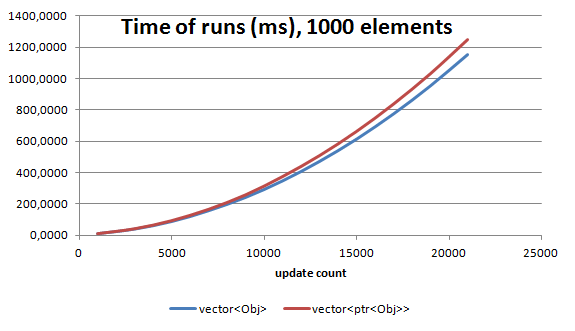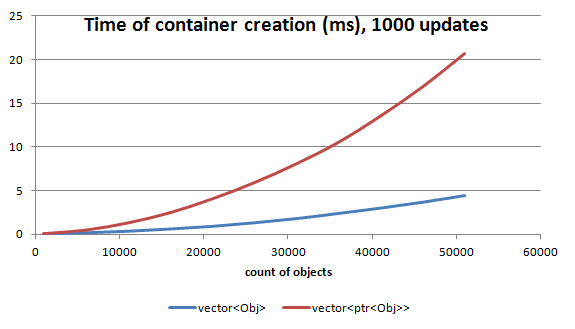# Vector of object vs Vector of pointers

See my new website at cppstories.com

After watching some of the talks from Build 2014 - especially "Modern C++: What You Need to Know" and some talks from Eric Brumer I started thinking about writing my own test case. Basically I've created simple code that compares `vector<Obj>` vs `vector<shared_ptr<Obj>>` The first results are quite interesting so I thought it is worth to describe this on the blog.

## Introduction

In the mentioned talks there was a really strong emphasis on writing memory efficient code. Only when you have nice memory access patterns you can reach maximum performance from your CPU. Of course one can use fancy CPU instructions, but they will not do much when code basically waits for the memory package to arrive.
I've compared the following cases:
• `std::vector<Object>` - memory is allocated on the heap but vector guarantees that the mem block is continuous. Thus, iteration over it should be quite fast.• `std::vector<std::shared_ptr<Object>>` - this simulates array of references from C#. You have an array, but each element is allocated in a different place in the heap. I wonder how much performance we loose when using such pattern. Or maybe it is not that problematic?## The code

As a more concrete example I've used Particle class.
Full repository can be found here: github/fenbf/PointerAccessTest

### Particle

``````class Particle
{
private:
float pos;
float acc;
float vel;
float col;
float rot;
float time;
``````
Generate method:
``````virtual void Particle::generate()
{
acc = randF();
acc = randF();
acc = randF();
acc = randF();
pos = pos = pos = pos = 0.0f;
vel = randF();
vel = randF();
vel = randF();
vel = vel + vel;
rot = 0.0f;
time = 1.0f+randF();
}
``````
Update method:
``````virtual void Particle::update(float dt)
{
vel += acc * dt;
vel += acc * dt;
vel += acc * dt;
vel += acc * dt;
pos += vel * dt;
pos += vel * dt;
pos += vel * dt;
pos += vel * dt;
col = pos * 0.001f;
col = pos * 0.001f;
col = pos * 0.001f;
col = pos * 0.001f;
rot += vel * dt;
time -= dt;

if (time < 0.0f)
generate();
}
``````

## The Test code

The test code:
• creates a desired container of objects
• runs generate method one time
• runs update method N times

### Vector of Pointers:

``````// start measuring time for Creation
std::vector<std::shared_ptr<Particle>> particles(count);
for (auto &p : particles)
{
p = std::make_shared<Particle>();
}
// end time measurment

for (auto &p : particles)
p->generate();

// start measuring time for Update
for (size_t u = 0; u < updates; ++u)
{
for (auto &p : particles)
p->update(1.0f);
}
// end time measurment
``````

### Vector of Objects:

``````// start measuring time for Creation
std::vector<Particle> particles(count);
// end time measurment

for (auto &p : particles)
p.generate();

// start measuring time for Update
for (size_t u = 0; u < updates; ++u)
{
for (auto &p : particles)
p.update(1.0f);
}
// end time measurment
``````

## The results

• Core i5 2400, Sandy Bridge
• Visual Studio 2013 for Desktop Express
• Release Mode
• /fp:fast, /arch:SSE2, /O2## Conclusion

• vector of shared pointers is around 8% slower (for 1000 of objects), but for larger number of objects in a container we can loose like 25%
• For small arrays and small number of updates/calls there is almost no difference. So if `shared_ptr` makes your code safer then it is better to use them. But still plain and simple array/container of Objects is preferred.
• For 50k of elements we spend 20ms on allocating memory for shared pointers!
• Vector of objects needs 5ms to allocate 50k though.
I need to finalize the code and maybe do some basic optimizations. Please let me know if something is wrong with the code!
Once again: repository can be found here: github/fenbf/PointerAccessTest

If you want to get additional C++ resources, exlusive articles, early access content, private Discord server and weekly curated news, check out my Patreon website: (see all benefits):